#### How to Calculate Complex Numbers in Excel

Performing calculations on complex numbers in Excel is not difficult because Excel has a set of functions that allow many different calculations of complex numbers.

Complex numbers consist of a real part and an imaginary part. You can perform arithmetic operations on complex numbers. Excel has built-in functions that will make it easier for you to perform complex calculations.

You can use a spreadsheet to perform operations with complex numbers. To use the complex number functions, you must first install the Analysis Toolpak.

`At this link you can learn how to add the Analysis Toolpak add-in.`

The functions that enable this are included in the group of engineering functions. Below we present the most important of them.

Wherever the argument is a complex number, enter it in the form “a + bi” or “a + bj”, where i and j denote the imaginary unit. This form can also be obtained in place of the argument by inserting the formula COMPLEX (a; b; “i”) or COMPLEX (a; b; “j”).

## How to insert a complex number

Use the COMPLEX function to create a complex number. The complex function has three arguments:

• real_num – this is the real part of a complex number
• i_num – imaginary part
• [suffix] – This is an optional argument. If it isn’t fixed, Excel will type i. Sometimes you may want to change to j.

The Complex formula =COMPLEX (5,7) creates a complex number 5 + 7i. 5 is the real part and 7 is the imaginary part.

## How to find the real part of a complex number

To find the real part of a complex number use the IMREAL function. The IMREAL function only takes one argument, which is inumber. In my case, using the formula = IMREAL (B3) will return 5 because 5 is the real part of the complex number 5 + 7i

## How to find the imaginary part of a complex number

Similarly, to find the imaginary part of a complex number, use the IMAGINARY function. The IMAGINARY function also takes only one argument to inumber. The formula = IMAGINARY (B3) will return 7.

## How to sum complex numbers

Use the IMSUM function to sum complex numbers. The syntax for the IMSUM function is = IMSUM (inumber1, inumber2, …). You must enter a minimum of two complex numbers that you want to add together.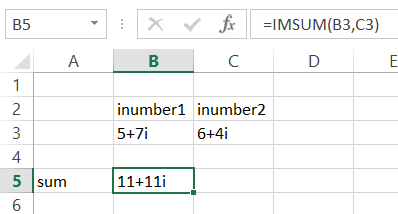The formula for adding complex numbers in my example is =IMSUM(B3,C3)

## Complex number subtraction

Use the IMSUB function to find the difference of complex numbers. The syntax for the IMSUB function is =IMSUB(inumber1, inumber2, …).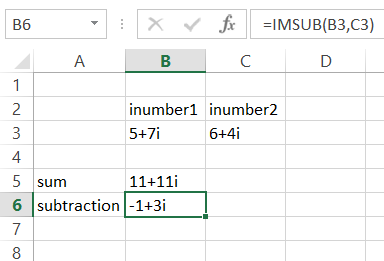The IMSUB formula I used: =IMSUB(B3,C3)

## How to find the product of complex numbers

Use the IMPRODUCT function to find the product of complex numbers.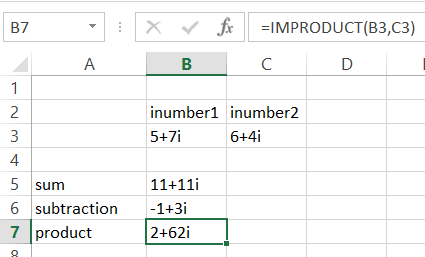In my case, the formula product to =IMPRODUCT(B3, C3) i gave the result 2 + 62i.

## How to find the quotient of complex numbers

Use the IMDIV function to find the quotient of complex numbers. The imdiv function returns the quotient of two complex numbers.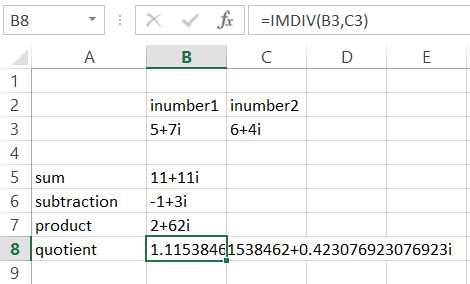In my case, the formula quotient is =IMDIV(B3, C3).

## How to find the absolute value of a complex number

Use the IMABS function to find the modulus of a complex number. The imabs function returns the absolute value of a complex number.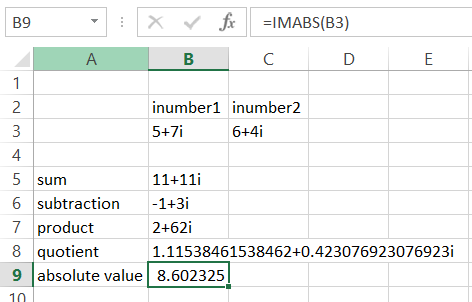In my case, the formula imabs is =IMABS(B3).

## How to find the argument of a complex number

To find the argument of a complex number, use the IMARGUMENT function. The imargument function returns the argument q of a complex number expressed in radians.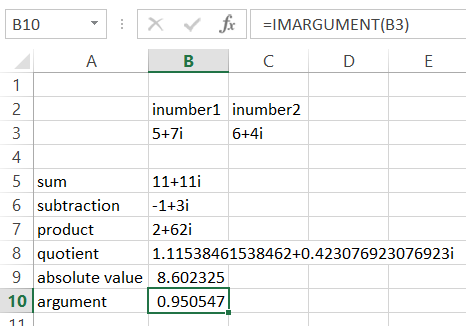In my case, the formula imabs is =IMARGUMENT(B3).

## How to find the conjugate of a complex number

Use the IMCONJUGATE function to find the conjugate of a complex number. The imargument function returns the conjugate of a complex number.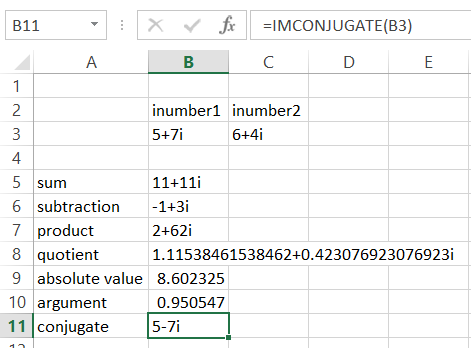In my case, the formula for imconjugate is =IMCONJUGATE(B3).

IMPOWER (team_number, integer_number) – returns a complex number raised to the power of a given integer.

## How to find the natural logarithm of a complex number

Use the IMLN function to find the natural logarithm of a complex number. The imargument function returns the natural logarithm of a complex number.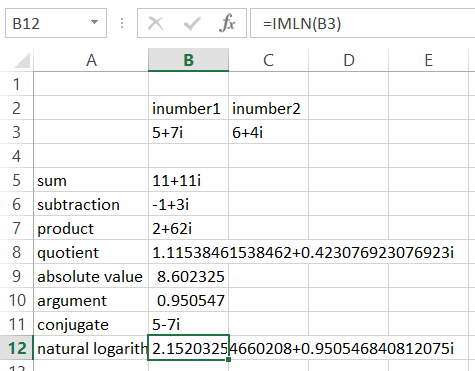In my case, the formula imln is =IMLN(B3).

Likewise, you can also calculate:

• base-10 logarithm of a complex number using the IMLOG10 function
• base-2 logarithm of a complex number using the IMLOG2 function

## How to find the square root of a complex number

Use the IMSQRT function to find the square root of a complex number. The imargument function returns the exp of a complex number.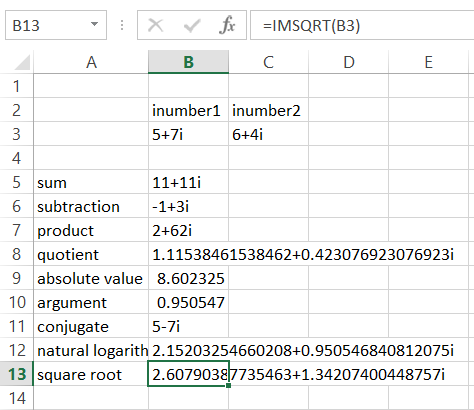In my case, the formula imexp is =IMSQRT(B3).

## How to find the exponential of a complex number

In order to find the exponents of a complex number use the IMEXP function. The imargument function returns the exp of a complex number.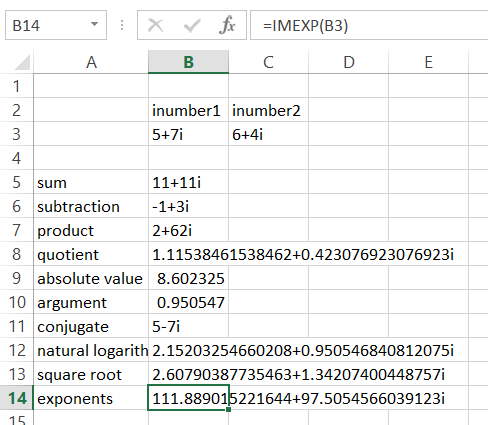In my case, the formula imexp is =IMEXP(B3).

The other complex functions:

• IMCOS function to calculate the cosine of a complex number
• IMCOSH function to calculate the hyperbolic cosine of a complex number
• IMCOT function to calculate the cotangent of a complex number
• IMCSC function to calculate the cosecant of a complex number
• IMCSCH function to calculate the hyperbolic cosecant of a complex number
• IMSEC function to calculate the secant of a complex number
• IMSECH function to calculate the hyperbolic secant of a complex number
• IMSIN function to calculate the sine of a complex number
• IMSINH function to calculate the hyperbolic sine of a complex number
• IMTAN function to calculate the tangent of a complex number

Hope the Excel tutorial of complex number calculator above helped you. I believe that thanks to this knowledge, no complex number calculations will be difficult for you.

## Key Takeaways

• Excel has a set of functions that allow many different calculations of complex numbers.
• The most important of these functions are COMPLEX, IMREAL, IMAGINARY, IMSUM, IMSUB, IMPRODUCT, IMDIV, IMABS, IMARGUMENT, IMCONJUGATE, IMLN, IMSQRT, and IMEXP.
• You can also use the IMCOS, IMCOSH, IMCOT, IMCSC, IMCSCH, IMSEC, IMSECH, IMSIN, IMSINH, and IMTAN functions to calculate the trigonometric functions of a complex number.

## FAQ

• Q: How do I install the Analysis Toolpak?
• A: You can install the Analysis Toolpak by going to File > Add-ins > Manage > Add-ins. In the Add-ins dialog box, select the Analysis Toolpak checkbox and click OK.
• Q: What is the difference between a real number and a complex number?
• A: A real number is a number that can be represented on a number line. A complex number is a number that can be represented as a combination of a real number and an imaginary number. The imaginary unit is denoted by i, and it is defined as the square root of -1.
• Q: What are some other uses for complex numbers?
• A: Complex numbers are used in many different areas of mathematics and science, including electrical engineering, physics, and chemistry. They are also used in some areas of finance and economics.